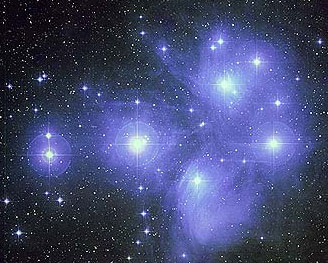The parallax angle is given in units of arcseconds. The distance to the star is given by the formula

`d = 1/p,`

where d is the distance to the star in parsecs (1 pc = 3.26 light-years) and p is the parallax angle in arc seconds.

Let’s practice. You can access a tool that shows an image of the sky with all Hipparcos data here (it will open in a new window). Read the directions on the page, then start the Java applet. Enter the coordinates given for Sirius and click on View. The applet will draw the region of sky around Sirius. The blue points are in the Hipparcos catalog and the Tycho catalog (objects in the Hipparcos catalog were measured with greater precision) and the white points are only in the Tycho catalog. The larger the circle, the brighter the star. Click on Sirius, the largest star in the middle, then click Get Info. Another window will open with a lot of information. You are most interested in line H11, Trigonometric parallax.

For Sirius, the parallax is 379.21 milliarcseconds. Plugging into our formula gives a distance of 2.637 parsecs. Converting to light-years gives a distance of 8.6 light-years.

Exercise 3. Use the Hipparcos data to find the distances to the following stars, given by RA and Dec. They are all in the same field you just called up to find Sirius. Record the Visual magnitudes of the stars as well. You will need them later.

RA Dec Visual Magnitude (Field H5) Parallax
(milli-arcsec)
Distance
(parsecs)
100.521 -15.209
99.648 -16.874
100.392 -17.534
102.203 -16.211

Record your data in this SkyServer workbook.

Now that you know these stars’ apparent visual magnitudes and distances, you can find their absolute magnitudes. Absolute magnitude is defined as the magnitude a star would appear to have if it were 10 parsecs away from us. Our Sun’s absolute magnitude is 4.84 (compared to its visible magnitude of -26.2!).

The relationship between a star’s apparent magnitude and absolute magnitude is given by the expression

`M = m - 5 log d + 5,`

where m is the star’s apparent magnitude, M is the star’s absolute magnitude, and d is the distance to the star in parsecs.

Let’s take the star Sirius again. It has a visual magnitude of -1.44 and it is 2.637 parsecs away. Therefore, its absolute magnitude is

`M = -1.44 - 5 log (2.637) + 5 = 1.45.`
Exercise 4: Use the visual magnitudes and distances you found earlier to find the absolute magnitudes of these stars.

RA Dec Visual Magnitude
(Field H5)
Distance Absolute
Magnitude
06 42 05 -15 12 32.8
06 38 35.43 -16 52 24.6
06 41 33.99 -17 32 01.0
06 48 48.78 -16 12 41.2

Now you are ready to make an H-R diagram!Copyright Anglo-Australian Observatory / Royal Observatory, Edinburgh
Courtesy AAO Astronomical Images Gallery.

Exercise 5. You will be making an H-R diagram using data from the Sky Plot tool you used earlier. Search around the Pleiades, a famous star cluster in the Northern Spring sky. Enter the appropriate coordinates and get your data. You will need the visual magnitude, parallax, and b-v color for each star. Get the data for many stars around the Pleiades. Construct an H-R diagram for the stars near the Pleiades.

Use this SkyServer workbook to keep track of your data. (HINT: You can enter use Excel to calculate the distance and absolute magnitude. It will save you a lot of calculation!)

Question 8. Based on their distances, which stars do you think belong to the Pleiades? Which stars just happen to be in the same part of the sky but are at different distances?
Question 9. Are most of the stars in the Pleiades main sequence stars, red giants, or white dwarfs?

You can use this information, in conjunction with the temperature of the star, to calculate the star’s radius. To learn how to calculate the radius of a star, click here.

One of the most important assumptions in science is that the laws of nature are the same everywhere in the universe. Your last two H-R diagrams suggest that this should be true – stars in the Pleiades show the same relationships as stars near the Sun.

But even including the Pleiades gives you H-R diagrams for stars only out to a distance of a few hundred light years. How can we look at stars farther away and see if they exhibit the same behavior? We cannot accurately measure distances to very distant stars because their parallax angles get too small.

When you looked at the Pleiades, you saw a star cluster. When you found the distance to each star, you found that they were all about the same. This finding makes sense: since the stars were together in a cluster, they should all be at about the same distance from Earth. The more distant the cluster, the safer it is to assume that all the stars are at the same distance.

If you assume that all the stars in a cluster are at the same distance, you can assume that each star’s observed apparent magnitude corresponds to its luminosity. In other words, you can make an H-R diagram using apparent magnitude instead of absolute magnitude. If you make an H-R diagram for a cluster, you don’t have to know the actual distance to the cluster. Therefore, you can make H-R diagrams for star clusters too far away to find distances with parallax. Star clusters are a powerful tool for making H-R diagrams.